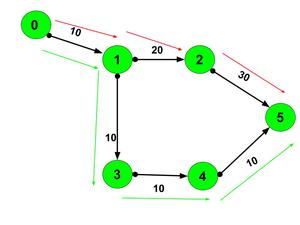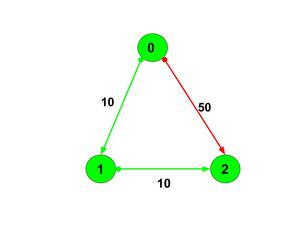# Minimum cost of path between given nodes containing at most K nodes in a directed and weighted graph

• Last Updated : 10 Feb, 2022

Given a directed weighted graph represented by a 2-D array graph[][] of size n and 3 integers src, dst, and k representing the source point, destination point, and the available number of stops. The task is to minimize the cost of the path between two nodes containing at most K nodes in a directed and weighted graph. If there is no such route, return -1.

Examples:

Input: n=6, graph[][] = [[0, 1, 10], [1, 2, 20], [1, 3, 10], [2, 5, 30], [3, 4, 10], [4, 5, 10]], src=0, dst=5, k=2
Output: 60
Explanation:Src = 0, Dst = 5 and k = 2

There can be a route marked with a green arrow that takes cost =  10+10+10+10=40 using three stops. And route marked with red arrow takes cost = 10+20+30=60 using two stops. But since there can be at most 2 stops, the answer will be 60.

Input: n=3, graph[][] = [[0, 1, 10], [0, 2, 50], [1, 2, 10], src=0, dst=2, k=1
Output:  20
Explanation:Src=0 and Dst=2

Since the k is 1, then the green-colored path can be taken with a minimum cost of 20.

Approach: Increase k by 1 because on reaching the destination, k+1 stops will be consumed. Use Breadth-first search to run while loop till the queue becomes empty. At each time pop out all the elements from the queue and decrease k by 1 and check-in their adjacent list of elements and check they are not visited before or their cost is greater than the cost of parent node + cost of that node, then mark their prices by prices[parent] + cost of that node. If k becomes 0 then break the while loop because either cost is calculated or we consumed k+1 stops. Follow the steps below to solve the problem:

• Increase the value of k by 1.
• Initialize a vector of pair adj[n] and construct the adjacency list for the graph.
• Initialize a vector prices[n] with values -1.
• Initialize a queue of pair q[].
• Push the pair {src, 0} into the queue and set the value of prices as 0.
• Traverse in a while loop until the queue becomes empty and perform the following steps:
• If k equals 0 then break.
• Initialize the variable sz as the size of the queue.
• Traverse in a while loop until sz is greater than 0 and perform the following tasks:
• Initialize the variables node as the first value of the front pair of the queue and cost as the second value of the front pair of the queue.
• Iterate over the range [0, adj[node].size()) using the variable it and perform the following tasks:
• If prices[it.first] equals -1 or cost + it.second is less than prices[it.first] then set the value of prices[it.first] as cost + it.second and push the pair {it.first, cost + it.second} into the queue.
• Reduce the value of k by 1.
• After performing the above steps, print the value of prices[dst] as the answer.

Below is the implementation of the above approach:

## C++

 `// C++ program for the above approach``#include ``using` `namespace` `std;` `// Function to find the minimum cost``// from src to dst with at most k stops``int` `findCheapestCost(``int` `n,``                     ``vector >& graph,``                     ``int` `src, ``int` `dst, ``int` `k)``{``    ``// Increase k by 1 Because on reaching``    ``// destination, k becomes k+1``    ``k = k + 1;` `    ``// Making Adjacency List``    ``vector > adj[n];` `    ``// U->{v, wt}``    ``for` `(``auto` `it : graph) {``        ``adj[it].push_back({ it, it });``    ``}` `    ``// Vector for Storing prices``    ``vector<``int``> prices(n, -1);` `    ``// Queue for storing vertex and cost``    ``queue > q;` `    ``q.push({ src, 0 });``    ``prices[src] = 0;` `    ``while` `(!q.empty()) {` `        ``// If all the k stops are used,``        ``// then break``        ``if` `(k == 0)``            ``break``;` `        ``int` `sz = q.size();``        ``while` `(sz--) {``            ``int` `node = q.front().first;``            ``int` `cost = q.front().second;``            ``q.pop();` `            ``for` `(``auto` `it : adj[node]) {``                ``if` `(prices[it.first] == -1``                    ``or cost + it.second``                           ``< prices[it.first]) {``                    ``prices[it.first] = cost + it.second;``                    ``q.push({ it.first, cost + it.second });``                ``}``            ``}``        ``}``        ``k--;``    ``}``    ``return` `prices[dst];``}` `// Driver Code``int` `main()``{``    ``int` `n = 6;``    ``vector > graph``        ``= { { 0, 1, 10 }, { 1, 2, 20 }, { 2, 5, 30 }, { 1, 3, 10 }, { 3, 4, 10 }, { 4, 5, 10 } };` `    ``int` `src = 0;``    ``int` `dst = 5;``    ``int` `k = 2;``    ``cout << findCheapestCost(n, graph, src, dst, k)``         ``<< endl;` `    ``return` `0;``}`

## Java

 `// Java program for the above approach``import` `java.util.*;` `class` `GFG{``  ``static` `class` `pair``  ``{``    ``int` `first, second;``    ``public` `pair(``int` `first, ``int` `second) ``    ``{``      ``this``.first = first;``      ``this``.second = second;``    ``}   ``  ``}``  ``// Function to find the minimum cost``  ``// from src to dst with at most k stops``  ``static` `int` `findCheapestCost(``int` `n,``                              ``int``[][] graph,``                              ``int` `src, ``int` `dst, ``int` `k)``  ``{``    ``// Increase k by 1 Because on reaching``    ``// destination, k becomes k+1``    ``k = k + ``1``;` `    ``// Making Adjacency List``    ``Vector []adj = ``new` `Vector[n];``    ``for` `(``int` `i = ``0``; i < adj.length; i++)``      ``adj[i] = ``new` `Vector();` `    ``// U.{v, wt}``    ``for` `(``int` `it[] : graph) {``      ``adj[it[``0``]].add(``new` `pair( it[``1``], it[``2``] ));``    ``}` `    ``// Vector for Storing prices``    ``int` `[]prices = ``new` `int``[n];``    ``Arrays.fill(prices, -``1``);` `    ``// Queue for storing vertex and cost``    ``Queue q = ``new` `LinkedList<>();` `    ``q.add(``new` `pair( src, ``0` `));``    ``prices[src] = ``0``;` `    ``while` `(!q.isEmpty()) {` `      ``// If all the k stops are used,``      ``// then break``      ``if` `(k == ``0``)``        ``break``;` `      ``int` `sz = q.size();``      ``while` `(sz-- >``0``) {``        ``int` `node = q.peek().first;``        ``int` `cost = q.peek().second;``        ``q.remove();` `        ``for` `(pair it : adj[node]) {``          ``if` `(prices[it.first] == -``1``              ``|| cost + it.second``              ``< prices[it.first]) {``            ``prices[it.first] = cost + it.second;``            ``q.add(``new` `pair( it.first, cost + it.second ));``          ``}``        ``}``      ``}``      ``k--;``    ``}``    ``return` `prices[dst];``  ``}` `  ``// Driver Code``  ``public` `static` `void` `main(String[] args)``  ``{``    ``int` `n = ``6``;``    ``int``[][] graph``      ``= { { ``0``, ``1``, ``10` `}, { ``1``, ``2``, ``20` `}, { ``2``, ``5``, ``30` `}, { ``1``, ``3``, ``10` `}, { ``3``, ``4``, ``10` `}, { ``4``, ``5``, ``10` `} };` `    ``int` `src = ``0``;``    ``int` `dst = ``5``;``    ``int` `k = ``2``;``    ``System.out.print(findCheapestCost(n, graph, src, dst, k)``                     ``+``"\n"``);` `  ``}``}` `// This code is contributed by 29AjayKumar`

## Python3

 `# python3 program for the above approach``from` `collections ``import` `deque` `# Function to find the minimum cost``# from src to dst with at most k stops``def` `findCheapestCost(n, graph, src, dst, k):` `    ``# Increase k by 1 Because on reaching``    ``# destination, k becomes k+1``    ``k ``=` `k ``+` `1` `    ``# Making Adjacency List``    ``adj ``=` `[[] ``for` `_ ``in` `range``(n)]` `    ``# U->{v, wt}``    ``for` `it ``in` `graph:``        ``adj[it[``0``]].append([it[``1``], it[``2``]])` `    ``# Vector for Storing prices``    ``prices ``=` `[``-``1` `for` `_ ``in` `range``(n)]` `    ``# Queue for storing vertex and cost``    ``q ``=` `deque()` `    ``q.append([src, ``0``])``    ``prices[src] ``=` `0` `    ``while` `(``len``(q) !``=` `0``):` `        ``# If all the k stops are used,``        ``# then break``        ``if` `(k ``=``=` `0``):``            ``break` `        ``sz ``=` `len``(q)``        ``while` `(``True``):``            ``sz ``-``=` `1` `            ``pr ``=` `q.popleft()` `            ``node ``=` `pr[``0``]``            ``cost ``=` `pr[``1``]` `            ``for` `it ``in` `adj[node]:``                ``if` `(prices[it[``0``]] ``=``=` `-``1``                        ``or` `cost ``+` `it[``1``]``                        ``< prices[it[``0``]]):``                    ``prices[it[``0``]] ``=` `cost ``+` `it[``1``]``                    ``q.append([it[``0``], cost ``+` `it[``1``]])` `            ``if` `sz ``=``=` `0``:``                ``break``        ``k ``-``=` `1` `    ``return` `prices[dst]` `# Driver Code``if` `__name__ ``=``=` `"__main__"``:` `    ``n ``=` `6``    ``graph ``=` `[``            ``[``0``, ``1``, ``10``],``            ``[``1``, ``2``, ``20``],``            ``[``2``, ``5``, ``30``],``            ``[``1``, ``3``, ``10``],``            ``[``3``, ``4``, ``10``],``            ``[``4``, ``5``, ``10``]]` `    ``src ``=` `0``    ``dst ``=` `5``    ``k ``=` `2``    ``print``(findCheapestCost(n, graph, src, dst, k))` `    ``# This code is contributed by rakeshsahni`

Output
`60`

Time Complexity: O(n*k)
Auxiliary Space: O(n)

My Personal Notes arrow_drop_up